Spitzer Documentation & Tools
IRS Instrument Handbook

### 5.1.11         LINEARIZ

The LINEARIZ (“Linearization”) module corrects the ramps of IRS DCEs for the non-linear response of the detectors. In the absence of this effect, the signal of a given sample, in a particular pixel (ramp), would ideally be a linear function of time from the beginning of the DCE, or equivalently of plane (sample) number counting from the first sample of the ramp.

An empirical, one-parametric model of non-linearity relates the observed signal Sobs of a sample of a given ramp, to the ideal signal Slin that would be observed in the absence of non-linearity: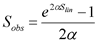Equation 5.9

The parameter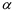is the so-called non-linearity coefficient. In the IRS pipelines,< 0, and its absolute value is || ~ 10-7 to 10-6 . Its units, 1/electron, are the reciprocal of the units of Slin or Sobs.

The above exponential model is used in SATCOR. A series expansion of the exponential, keeping terms up to second order, leads to the so-called quadratic model of non-linearity that is used in LINEARIZ: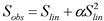Equation 5.10

The above can be solved for Slin as a function of Sobs; only one of the two quadratic solutions is valid, given the requirement that Sobs should approach Slin as α approaches 0: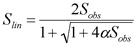Equation 5.11

The application of the above expression, given the input signal Sobs of a sample, and the non-linearity coefficientobtained from the lincal.fits calibration file, constitutes the main algorithm of the LINEARIZ module.

There are two conditions under which the module cannot correct a sample Sobs. In either case, a fatal bit is set in the output (updated) dmask.fits file for the corresponding sample.

-If Sobs is larger than a threshold for non-linearity read from the lincal.fits calibration file, the sample is deemed as “saturated beyond correctable non-linearity.” The threshold in current IRS pipelines is 280,000 electrons. IRS observations typically do not reach this threshold without also being digitally saturated. Bit # 13 is set in the dmask.fits corresponding sample.

-If Sobs> |1/4|, the solution for Slin is imaginary. In such case, it is deemed that linearity could not be applied for the particular sample. Bit # 12 is set in the corresponding dmask.fits sample.

The calibration file lincal.fits is a 3-plane cube. Its first plane contains thecoefficient for each of the 16,384 pixels in IRS arrays. The same value ofis used for all pixels of a given instrument channel. The second plane contains the threshold for saturation beyond correctable non-linearity, for each pixel. The third plane is the uncertainty in, also for each pixel. This uncertainty is propagated in the BCD pipeline.

The outputs of this module are lnz.fits and lnzu.fits.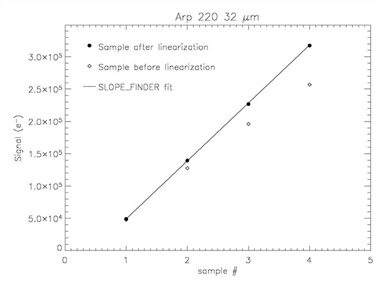Figure 5.5  Illustration of non-linearity correction in a LL 6 second ramp for a bright source: the open rhombii are input samples, referred to as Sobs in the text; the filled circles are output samples, referred to as Slin, after the operation of LINEARIZ. The solid line is the linear fit computed by the subsequent module SLOPE_FINDER.